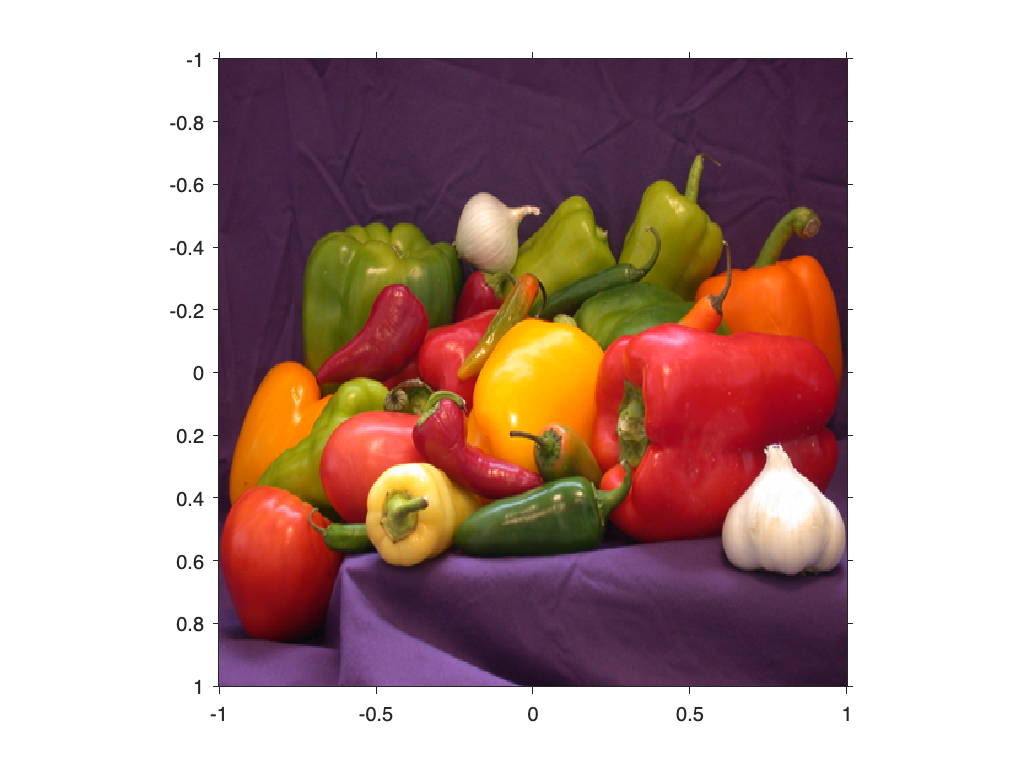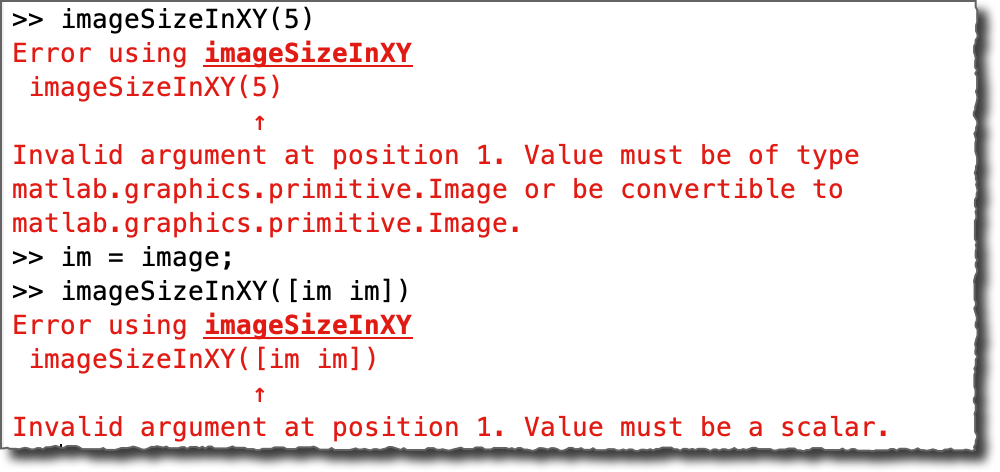# An Example of Function Argument Validation for an Image Utility Function

While I was working on a prototype yesterday, I found myself writing a small bit of code that turned out to be so useful, I wondered why I had never done it before. I'd like to show it to you. It'll be an opportunity to explore some recent advances in MATLAB that make useful function syntaxes easy to write.
I wrote a simple function called imageSizeInXY. This function takes a MATLAB Graphics Image object and returns its total width and height in the spatial x-y units of the axes.
function out = imageSizeInXY(im)

...

end
(I'll show the full implementation below.) I was using the function like this.
im = imshow(A, XData = [-1 1], YData = [-1 1]);
axis onimageSizeInXY_v1(im)
ans = 1×2
2.0039 2.0052
But this function only works if I have captured the image object in a variable. Usually, I call imshow with no output argument, and so I don't have the image object:
imshow(A, XData = [-1 1], YData = [-1 1])
I thought to myself, it would sure be nice if I could just call imageSizeInXY with no input arguments, and it would just automatically tell me about the image that's displayed in the current figure. After thinking about this briefly, I wrote the following:
function out = imageSizeInXY(im)
arguments
im (1,1) matlab.graphics.primitive.Image = findimage
end

...

end

function im = findimage
image_objects = imhandles(imgca);
if isempty(image_objects)
error("No image object found.");
end
im = image_objects(1);
end
The first interesting piece here is the use of the arguments block, a MATLAB language syntax that was introduced in R2019b. The arguments block, and more generally the function argument validation features, helps to implement function behavior with helpful error messages and useful defaults while writing very little code.
Let's look more closely at the beginning of the function.
function out = imageSizeInXY(im)
arguments
im (1,1) matlab.graphics.primitive.Image = findimage
end
The arguments block lets us specify constraints and default behavior for the function's input arguments. The "(1,1)" part says that the input argument, im, must have size $1 \times 1$. In other words, it must be a scalar. The next part, "matlab.graphics.primitive.Image," indicates that im is required to be that class (or convertible to it).
MATLAB will enforce these size and type constraints automatically, issuing specific error messages if they are not met.Note that you can get the full class name of MATLAB graphics objects by calling class:
class(im)
ans = 'matlab.graphics.primitive.Image'
The "= findimage" portion is used to automatically provide a default value for the input im. This can be a constant value or an expression. In this case, findimage is an expression that calls the local function that I have written:
function im = findimage
image_objects = imhandles(imgca);
if isempty(image_objects)
error("No image object found.");
end
im = image_objects(1);
end
The functions imgca and imhandles are Image Processing Toolbox utilities. The function imgca returns the most recently current axes object containing one or more images, and imhandles returns the image objects within it.
The last few lines are just me being careful to handle the cases where there are no image objects to be found, or where there are multiple image objects in the axes.
The full implementation, including the simple size calculation, is below.
function out = imageSizeInXY(im)
arguments
im (1,1) matlab.graphics.primitive.Image = findimage
end

pixel_width = extent(im.XData,size(im.CData,2));
pixel_height = extent(im.YData,size(im.CData,1));

x1 = im.XData(1) - (pixel_width / 2);
x2 = im.XData(end) + (pixel_width / 2);

y1 = im.YData(1) - (pixel_height / 2);
y2 = im.YData(end) + (pixel_height / 2);

out = [(x2 - x1) (y2 - y1)];
end

function im = findimage
image_objects = imhandles(imgca);
if isempty(image_objects)
error("No image object found.");
end
im = image_objects(1);
end

function e = extent(data,num_pixels)
if (num_pixels <= 1)
e = 1;
else
e = (data(2) - data(1)) / (num_pixels - 1);
end
end
Now I don't have to remember to call imshow with an output argument in order to use it.
If you haven't already explored using function argument validation and arguments blocks for writing your own MATLAB functions, I encourage you to give them a try. It's really worth it!
|

### コメント

コメントを残すには、ここ をクリックして MathWorks アカウントにサインインするか新しい MathWorks アカウントを作成します。